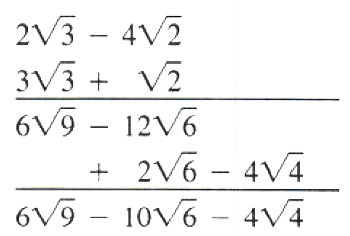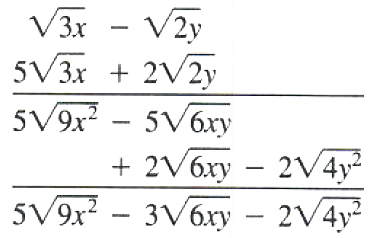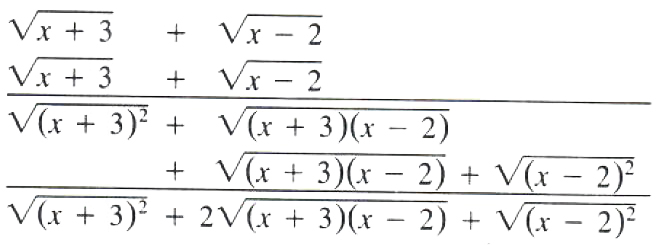Algebra

Equations

Inequalities

Graphs

Numbers

Calculus

Matrices

Tutorials

# Multiplication and Division of Radical Expressions

Multiplication of radical expressions is possible using the rule

na nb=nab for a,bR,a>0,b>0

EXAMPLE  1. 2 3=2·3=6

2. 6 33=6·33

=2·3·3·11=3 22

3. 2 x xy=2 x·xy=2 x2 y=2 xy

4. 3 32·2 35=(3·2) 32·5=6 310

5. 34 36=324=323·3=2 33

Note    The final radical must be in standard form.

To multiply one radical by a radical expression of more than one term, we use the distributive law: a (b+c)=ab+ac.

EXAMPLE  Multiply 3 2 (5 6-2 10) and simplify.

Solution   3 2 (5 6-2 10)

=3 2·5 6-3 2·2 10

=12-6 20

=30 3-12 5

EXAMPLE  Multiply 2 3 xy (4 x-3 y) and simplify.

Solution   2 3 xy (4 x-3 y)

=8 3 x2 y-6 3 xy2

=8 x3 y-6 y3 x

To multiply two radical expressions, each with more than one term, follow the same arrangement as in multiplying polynomials.

EXAMPLE  Multiply (2 3-4 2) by (3 3+2) and simplify.

SolutionHence (2 3-4 2) (3 3+2)

=6 9-10 6-4 4

=18-10 6-8

=10-10 6

EXAMPLE  Multiply 3 x-2 y by 5 3 x+2 2 y and simplify.

SolutionHence (3 x-2 y)(5 3 x+2 2 y)

=5 9 x2-3 6 xy-2 4 y2

=15 x-3 xy-4 y

=15 x-4 y-3 6 xy

EXAMPLE  Expand (x+3+x-2)2 and simplify.

SolutionHence (x+3+x-2)2

=(x+3)2+2 (x+3) (x-2)+(x-2)2

=x+3+2 x2+x-6+x-2

=2 x+1+2 x2+x-6

Let’s see how our math solver simplifies this and similar problems. Click on "Solve Similar" button to see more examples.

Note     (a+b)2a+b

(a+b)2=(a+b) (a+b)=a+2 ab+b  When the radicals have different indices, we apply the rule nam=nkamkto make the indices the same as their LCM and then apply na nb=nab.

EXAMPLES  1. 3 332=633 637=637=3 63

2. 3a2 4a3=12a8 12a9=12a17=a12a5

THEOREM  When a,bR,a>0,b>0, and nN, then nanb=nab.

Proof    nanb=a1nb1n=(ab)1n=nab

Radical expressions can be divided according to the above theorem only when the radical indices are the same. For different radical indices, the preliminary step to make them the same must be carried out.

EXAMPLES  1. 155=155=3

2. x3 y5x2 y=x3 y5x2 y=xy4=y2 x

Sometimes the numerator of a fractional radicand is not an exact multiple of the denominator, for example 32 To simplify such a radical, multiply both numerator and denominator of the radicand by the smallest number that will make the denominator a perfect root.

Note    The denominator is a perfect root if the exponent of each factor is an integral multiple of the radical index.

To simplify 32multiply the numerator and denominator of the radicand by 2.

32=3·22·2=62 or 126

It is easier to manipulate 126 than 32.

Remark  When the radical expression is of the form ab sqrt (c), multiply the numerator and the denominator by c.

23=23=33=2 33

450=45 2=45 2=22=210=2 25

EXAMPLE  Divide 15 by 21and put in standard form.

Solution   1521=1521=57=5·77·7=17 35

EXAMPLE  Divide 3 xy by 4 a3 band put in standard form.

Solution   3 xy4 a3 b=3 xy22 a3 b=3 xy22 a4 b·abab

=3 xyab22 a4 b2

=12 a2 b 3 xyab

EXAMPLE  Put 3 a2 b320 xy5in standard form.

Solution   3 a2 b320 xy5=3 a2 b322·5 xy5·5 xy5 xy

=15 a2 b3 xy22·52 x2 y6

=ab10 xy3 15 bxy

EXAMPLE  Divide 33 by 320and put in standard form.

Solution   33320=3322·5=3322·5·2·522·52

=33·2·5223·53

=110 3150

EXAMPLE  Put 381 x6 y78 a8 b10in standard form.

Solution   381 x6 y78 a8 b10=334 x6 y723 a8 b10=334 x6 y723 a8 b10·ab2ab2

=334 x6 y7 ab223 a9 b12=3 x2 y22 a3 b4 33 yab2

The deﬁnition of addition of fractions, a+bc=ac+bc, is used to divide a radical expression with more than one term by a one-term radical.

EXAMPLE  Divide and simplify 3 6-6 103 2.

Solution   3 6-6 103 2

=3 63 2-6 103 2

=62-2 102

=62-21 102

=3-2 5

EXAMPLE  Divide and simplify 7 x-2 y14 xy.

Solution   7 x-2 y14 xy

=7 x14 xy-2 y14 xy

=7 x14 xy-2 y14 xy

=12 y-17 x

=2 y22 y2-7 x72 x2

=12 y 2 y-17 x 7 x

When we multiply the radical expressions (a+b) and (a-b), we have get the rational expression (a-b). Each of the expressions (a+b) and (a-b) is called a rationalizing factor of other.

EXAMPLES  1. 2-3is a rationalizing factor of 2+3.

2. 2+3 2is a rationalizing factor of 2-3 2.

To facilitate the manipulation with a radical expression such as 2+32 2+3, we change the fraction to an equivalent one with a rational denominator. This can be accomplished by multiplying both numerator and denominator by the rationalizing factor of the denominator, 2 2-3.

This operation is called rationalizing the denominator

EXAMPLE  Rationalize the denominator of 22-3.

Solution   22-3

=2 (2+3)(2-3) (2+3)

=2 2+64-3=2 2+6

EXAMPLE  Rationalize the denominator of 2+32 2+3.

Solution   2+32 2+3

=(2+3) (2 2-3)(2 2+3) (2 2-3)

=1+68-3

=15 (1+6)

Let’s see how our math solver simplifies this and similar problems. Click on "Solve Similar" button to see more examples.

 ← Previous Tutorial Next Tutorial →